# Some notes on PChange – estimating when trajectories cross over time

J.C. Barnes and company published a paper in JQC not too long ago and came up with a metric, PChange, to establish the number of times trajectories cross in a sample. This is more of interest to life course folks, although it is not totally far fetched to see it applied to trajectories of crime at places. Part of my interest in it was simply that it is an interesting statistical question — when two trajectories with errors cross. A seemingly simple question that has a few twists and turns. Here are my subsequent notes on that metric.

# The Domain Matters

First, here is an example of the trajectories not crossing: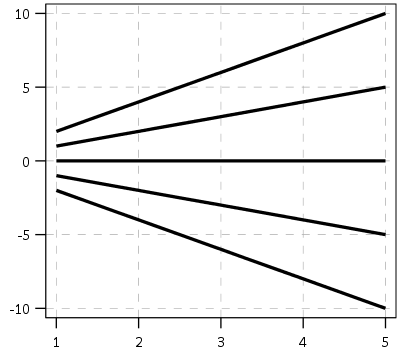This points to an important assumption about those lines not crossing though that was never mentioned in the Barnes paper — the domain matters. For instance, if we draw those rays further back in time what happens?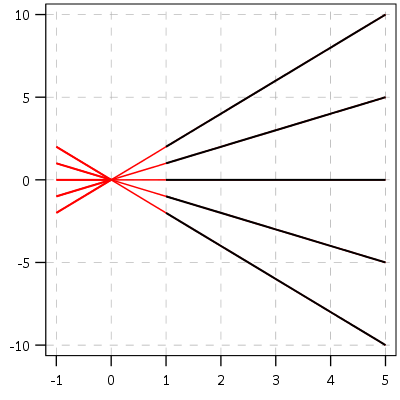They cross! This points to an important piece of information when evaluating PChange — the temporal domain in which you examine the data matters. So if you have a sample of juvenile delinquency measures from 14-18 you would find less change than a similar sample from 12-20.

This isn’t really a critique of PChange — it is totally reasonable to only want to examine changes within a specific domain. Who cares if delinquency trajectories cross when people are babies! But it should be an important piece of information researchers use in the future if they use PChange — longer samples will show more change. It also won’t be fair to compare PChange for samples of different lengths.

# A Functional Approach to PChange

For above you may ask — how would you tell if a trajectory crosses outside of the domain of the data? The answer to that question is you estimate an underlying function of the trajectory — some type of model where the outcome is a function of age (or time). With that function you can estimate the trajectory going back in time or forward in time (or in between sampled measurements). You may not want to rely on data outside of the domain (its standard error will be much higher than data within the time domain, forecasting is always fraught with peril!), but the domain of your sample is ultimately arbitrary. So what about the question will the trajectories ever cross? Or would the trajectories have crossed if I had data for ages 12-20 instead of just 16-18? Or would they have crossed if I checked the juveniles at age 16 1/2 instead of only at 16?

So actually instead of the original way the Barnes paper formulated PChange, here is how I thought about calculating PChange. First you estimate the underlying trajectory for each individual in your sample, then you take the difference of those trajectories.

``````y_i = f(t)
y_j = g(t)
y_delta = f(t) - g(t) = d(t)``````

Where `y_i` is the outcome `y` for observation `i`, and `y_j` is the outcome `y` for observation `j`. `t` is a measure of time, and thus the anonymous functions `f` and `g` represent growth models for observations i and j over time. `y_delta` is then the difference between these two functions, which I represent as the new function `d(t)`. So for example the functions for each individual might be quadratic in time:

``````y_i = b0i + b1i(t) + b2i(t^2)
y_j = b0j + b1j(t) + b2j(t^2)``````

Subsequently the difference function will also be quadratic, and can be simply represented as:

``y_delta = (b0i - b0j) + (b1i - b1j)*t + (b2i - b2j)*t^2``

Then for the trajectories to cross (or at least touch), `y_delta` just then has to equal zero at some point along the function. If this were math, and the trajectories had no errors, you would just set d(t) = 0 and solve for the roots of the equation. (Most people estimating models like these use functions that do have roots, like polynomials or splines). If you cared about setting the domain, you would then just check if the roots are within the domain of interest — if they are, the trajectories cross, if they are not, then they do not cross. For data on humans with age, obviously roots for negative human years will not be of interest. But that is a simple way to solve the domain problem – if you have an underlying estimate of the trajectory, just see how often the trajectories cross within equivalent temporal domains in different samples.

I’d note that the idea of having some estimate of the underlying trajectory is still relevant even within the domain of the data — not just extrapolating to time periods outside. Consider two simple curves below, where the points represent the time points where each individual was measured.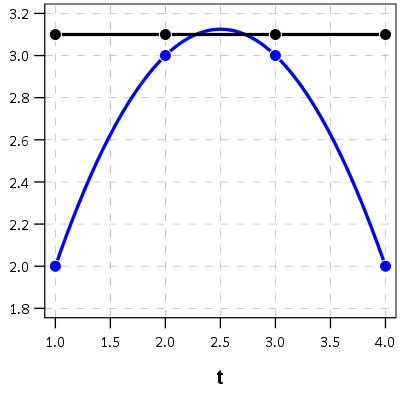So while the two functions cross, when only considering the sampled locations, like is done in Barnes et al.’s PChange, you would say these trajectories do not cross, when in actuality they do. It is just the sampled locations are not at the critical point in the example for these two trajectories.

This points to another piece of contextual information important to interpreting PChange — the number of sample points matter. If you have samples every 6 months, you will likely find more changes than if you just had samples every year.

I don’t mean here to bag on Barnes original metric too much — his PChange metric does not rely on estimating the underlying functional form, and so is a non-parametric approach to identifying change. But estimating the functional form for each individual has some additional niceties — one is that you do not need the measures to be at equivalent sample locations. You can compare someone measured at 11, 13, and 18 to someone who is measured at 12, 16, and 19. For people analyzing stuff for really young kids I bet this is a major point — the underlying function at a specific age is more important then when you conveniently measured the outcome. For older kids though I imagine just comparing the 12 to 11 year old (but in the same class) is probably not a big deal for delinquency. It does make it easier though to compare say different cohorts in which the measures are not at nice regular intervals (e.g. Add Health, NLYS, or anytime you have missing observations in a longitudinal survey).

In the end you would only want to estimate an underlying functional form if you have many measures (more so than 3 in my example), but this typically ties in nicely with what people modeling the behavior over time are already doing — modeling the growth trajectories using some type of functional form, whether it is a random effects model or a group based trajectory etc., they give you an underlying functional form. If you are willing to assume that model is good enough to model the trajectories over time, you should think it is good enough to calculate PChange!

# The Null Matters

So this so far would be fine and dandy if we had perfect estimates of the underlying trajectories. We don’t though, so you may ask, even though `y_delta` does not exactly equal zero anywhere, its error bars might be quite wide. Wouldn’t we then still infer that there is a high probability the two trajectories cross? This points to another hidden assumption of Barnes PChange — the null matters. In the original PChange the null is that the two trajectories do not cross — you need a sufficient change in consecutive time periods relative to the standard error to conclude they cross. If the standard error is high, you won’t consider the lines to cross. Consider the simple table below:

``````Period A_Level A_SE B_Level B_SE
1      4         1    1.5   0.5
2      5         1    3     0.5
3      6         1    4.5   0.5
4      7         1    6     0.5``````

Where A_Level and B_Level refer to the outcome for the four time periods, and A_SE and B_SE refer to the standard errors of those measurements. Here is the graph of those two trajectories, with the standard error drawn as areas for the two functions (only plus minus one standard error for each line).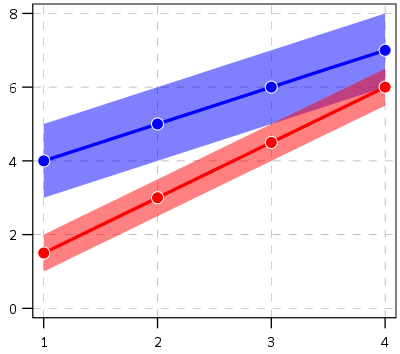And here is the graph of the differences — assuming that the covariance between the two functions is zero (so the standard error of the difference equals `sqrt(A_SE^2 + B_SE^2)`). Again only plus/minus one standard error.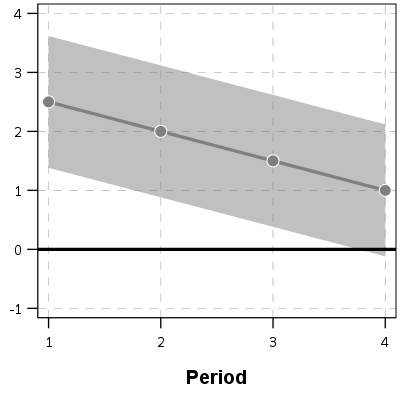You can see that the line never crosses zero, but the standard error area does. If our null is `H0: y_delta = 0 for any t`, then we would fail to reject the null in this example. So in Barnes original PChange these examples lines would not cross, whereas with my functional approach we don’t have enough data to know they don’t cross. This I suspect would make a big difference in many samples, as the standard error is going to be quite large unless you have very many observations and/or very many time points.

If one just wants a measure of crossed or did not cross, with my functional approach you could set how wide you want to draw your error bars, and then estimate whether the high or low parts of that bar cross zero. You may not want a discrete measure though, but a probability. To get that you would integrate the probability over the domain of interest and calculate the chunk of the function that cross zero. (Just assume the temporal domain is uniform across time.)

So in 3d, my difference function would look like this, whereas to the bottom of the wall is the area to calculate the probability of the lines crossing, and the height of the surface plot is the PDF at that point. (Note the area of the density is not normalized to sum to 1 in this plot.)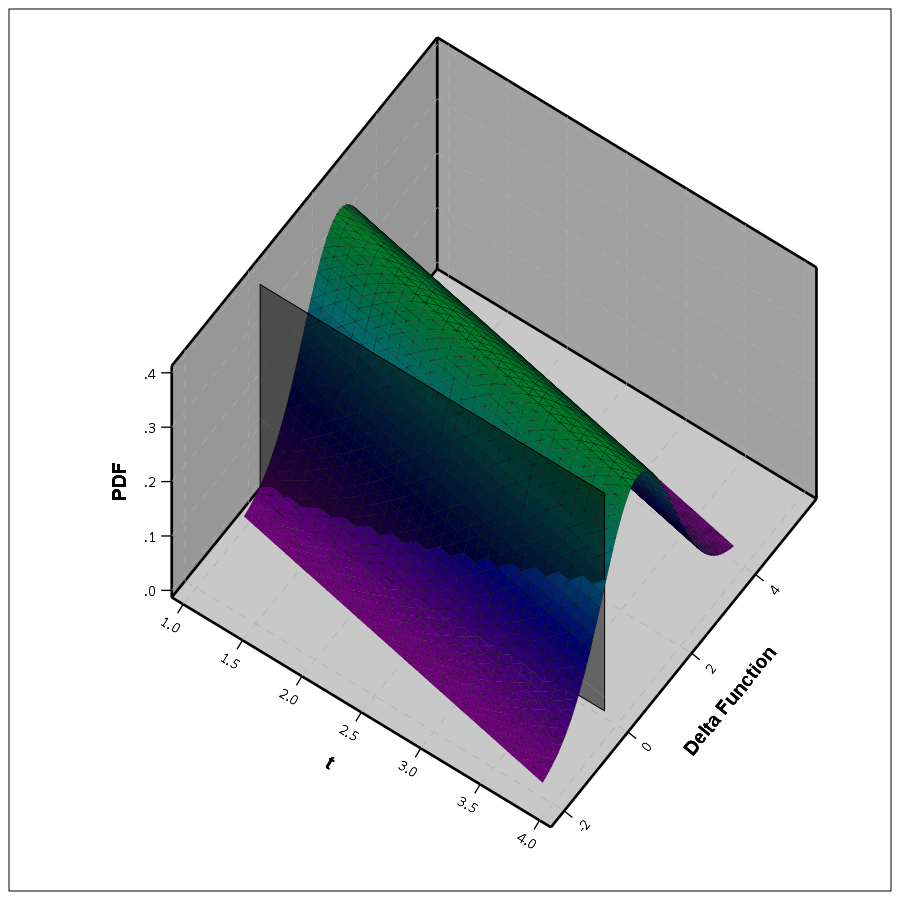This surface graph ends up crossing more than was observed in my prior 2d plots, as I was only plotting 1 standard error. Here imagine that the top green part of the density is the mean function — which does not cross zero — but then you have a non-trivial amount of the predicted density that does cross the zero line.

In the example where it just crosses one time by a little, it seems obvious to consider the small slice as the probability of the two lines crossing. I think to extend this to not knowing to test above or below the line you could calculate the probability on either side of the line, take the minimum, and then double that minimum for your p-value. So if say 5% of the area is below the line in my above example, you would double it and say the two-tailed p-value of the lines crossing is p = 0.10. Imagine the situation in which the line mostly hovers around 0, so the mass is about half on one side and half on the other. In that case the probability the lines cross seems much higher than 50%, so doubling seems intuitively reasonable.

So if you consider this probability to be a p-value, with a very small p-value you would reject the null that the lines cross. Unlike most reference distributions for p-values though, you can get a zero probability estimate of the lines crossing. You can aggregate up those probabilities as weights when calculating the overall PChange for the sample. So you may not know for certain if two trajectories cross, but you may be able to say these two trajectories cross with a 30% probability.

Again this isn’t to say that PChange is bad — it is just different. I can’t give any reasoning whether assuming they do cross (my functional approach) or assuming they don’t cross (Barnes PChange) is better – they are just different, but would likely make a large difference in the estimated number of crossings.

# Population Change vs Individual Level Change

So far I have just talked about trying to determine whether two individual lines cross. For my geographic analysis of trajectories in which I have the whole population (just a sample in time), this may be sufficient. You can calculate all pairwise differences and then calculate PChange (I see no data based reason to use the permutation sample approach Barnes suggested – we don’t have that big of samples, we can just calculate all pairwise combinations.)

But for many of the life course researchers, they are more likely to be interested in estimating the population of changes from the samples. Here I will show how you can do that for either random effects models, or for group based trajectory models based on the summary information. This takes PChange from a sample metric to a population level metric implied via your models you have estimated. This I imagine will be much easier to generalize across samples than the individual change metrics, which will be quite susceptible to outlier trajectories, especially in small samples.

First lets start with the random effects model. Imagine that you fit a linear growth model — say the random intercept has a variance of 2, and the random slope has a variance of 1. So these are population level metrics. The fixed effects and the covariance between the two random effect terms will be immaterial for this part, as I will discuss in a moment.

First, trivially, if you selected two random individuals from the population with this random effects distribution, the probability their underlying trajectories cross at some point is 1. The reason is for linear models, two lines only never cross if the slopes are perfectly parallel. Which sampling from a continuous random distribution has zero probability of them being exactly the same. This does not generalize to more complicated functions (imagine parabolas concave up and concave down that are shifted up and down so they never cross), but should be enough of a motivation to make the question only relevant for a specified domain of time.

So lets say that we are evaluating the trajectories over the range `t = [10,20]`. What is the probability two individuals randomly sampled from the population will cross? So again with my functional difference approach, we have

``````y_i = b0i + b1i*t
y_j = b0j + b1j*t
y_delta = (b0i - b0j) + (b1i - b1j)*t``````

Where in this case the `b0` and `b1` have prespecified distributions, so we know the distribution of the difference. Note that in the case with no covariates, the fixed effects will cancel out when taking the differences. (Generalizing to covariates is not as straightforward, you could either assume they are equal so they cancel out, or you could have them vary according to additional distributions, e.g. males have an 90% chance of being drawn versus females have a 10% chance, in that case the fixed effects would not cancel out.) Here I am just assuming they cancel out. Additionally, taking the difference in the trajectories also cancels out the covariance term, so you can assume the covariance between `(b0i - b0j)` and `(b1i - b1j)` is zero even if `b0` and `b1` have a non-zero covariance for the overall model. (Post is long enough — I leave that as an exercise for the reader.)

For each of the differences the means will be zero, and the variance will be the two variances added together, e.g. `b0i - b0j` will have a mean of zero and a variance of `2 + 2 = 4`. The variance of the difference in slopes will then be 2. Now to figure out when the two lines will cross.

If you make a graph where the X axis is the difference in the intercepts, and the Y axis is the difference in the slopes, you can then mark off areas that indicate the two lines will cross given the domain. Here for example is a sampling of where the lines cross – red is crossing, grey is not crossing.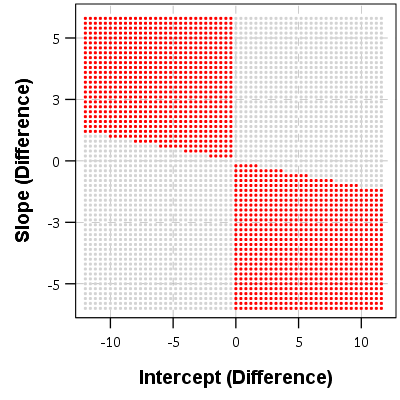So for example, say we had two random draws:

``````y_i = 1   + 0.5*t
y_j = 0.5 + 0.3*t
y_delta = 0.5 + 0.2*t``````

This then shows that the two lines do not cross when only evaluating `t` between 10 and 20. They have already diverged that far out (you would need negative `t` to have the lines cross). Imagine if `y_delta = -6 + 0.2*t` though, this line does cross zero though, at `t = 10` this function equals -1, whereas at `t = 20` the function equals 4.

If you do another 3d plot you can plot the bivariate PDF. Again integrate the chunks of areas in which the function crosses zero, and voila, you get your population estimate.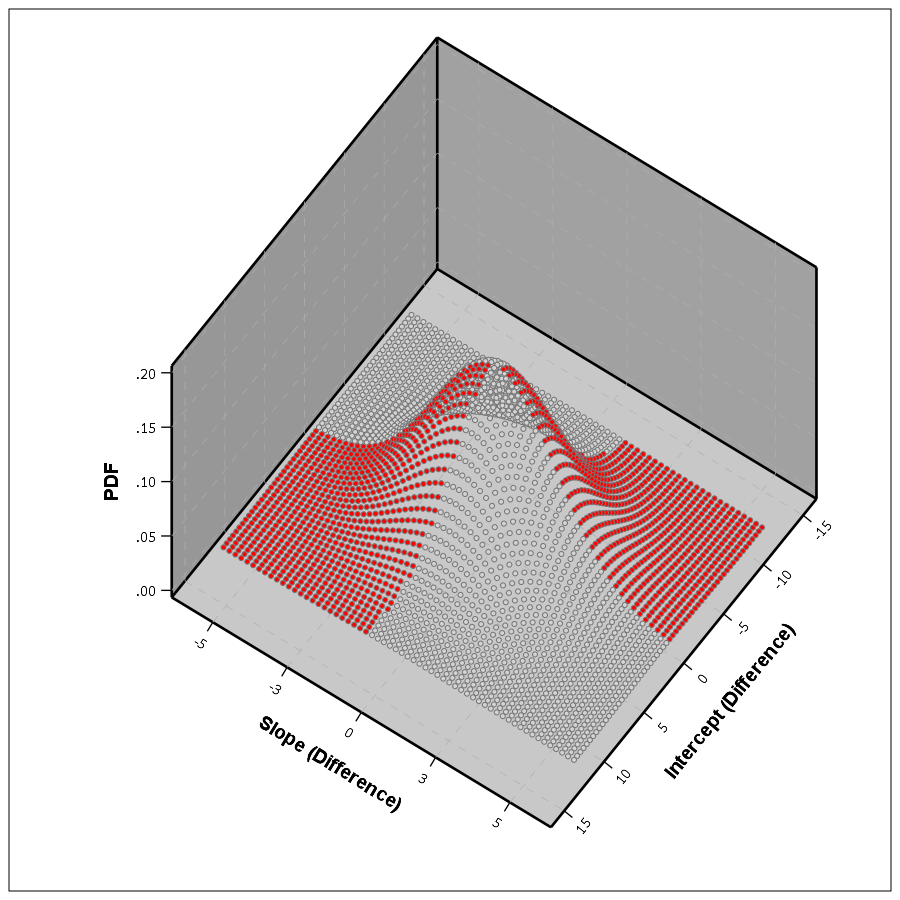This works in a similar manner to higher order polynomials, but you can’t draw it in a nice graph though. I’m blanking at the moment of a way to find these areas offhand in a nice way — suggestions welcome!

This gets a bit tricky thinking about in relation to individual level change. This approach does not assume any error in the random draws of the line, but assumes the draws will have a particular distribution. So the PChange does not come from adding up whether individual lines in your sample cross, it comes from the estimated distribution of what the difference in two randomly drawn lines would look like that is implied by your random effects model. Think if based on your random effect distribution you randomly drew two lines, calculated if they crossed, and then did this simulation a very large number of times. The integrations I’m suggesting are just an exact way to calculate PChange instead of the simulation approach.

If you were to do individual change from your random effects model you would incorporate the standard error of the estimated slope and intercept for the individual observation. This is for your hypothetical population though, so I see no need to incorporate any error.

Estimating population level change from group based trajectory models via my functional approach is more straightforward. First, with my functional approach you would assume individuals who share the same latent trajectory will cross with a high probability, no need to test that. Second, for testing whether two individual trajectories cross you would use the approach I’ve already discussed around individual lines and gain the p-value I mentioned.

So for example, say you had a probability of 25% that a randomly drawn person from group A would cross a randomly drawn person from Group B. Say also that Group A has 40/100 of the sample, and Group B is 60/100. So then we have three different groups: A to A, B to B, and A to B. You can then break down the pairwise number in each group, as well as the number of crosses below.

``````Compare   N    %Cross Cross
A-A      780    100    780
B-B     1770    100   1770
A-B     2400     25    600
Total   4950     64   3150``````

So then we have a population level p-change estimate of 64% from our GBTM. All of these calculations can be extended to non-integers, I just made them integers here to simplify the presentation.

Now, that overall PChange estimate may not be real meaningful for GBTM, as the denominator includes pairwise combinations of folks in the same trajectory group, which is not going to be of much interest. But just looking at the individual group solutions and seeing what is the probability they cross could be more informative. For example, although Barnes shows the GBTM models in the paper as not crossing, depending on how wide the standard errors of the functions are (that aren’t reported), this functional approach would probably assign non-zero probability of them crossing (think low standard error for the higher group crossing a high standard error for the low group).

Phew — that was a long post! Let me know in the comments if you have any thoughts/concerns on what I wrote. Simple question — whether two lines cross — not a real simple solution when considering the statistical nature of the question though. I can’t be the only person to think about this though — if you know of similar or different approaches to testing whether two lines cross please let me know in the comments.

# Graphs and interrupted time series analysis – trends in major crimes in Baltimore

Pete Moskos’s blog is one I regularly read, and a recent post he pointed out how major crimes (aggravated assaults, robberies, homicides, and shootings) have been increasing in Baltimore post the riot on 4/27/15. He provides a series of different graphs using moving averages to illustrate the rise, see below for his initial attempt:He also has an interrupted moving average plot that shows the break more clearly – but honestly I don’t understand his description, so I’m not sure how he created it.I recreated his initial line plot using SPSS, and I think a line plot with a guideline shows the bump post riot pretty clearly.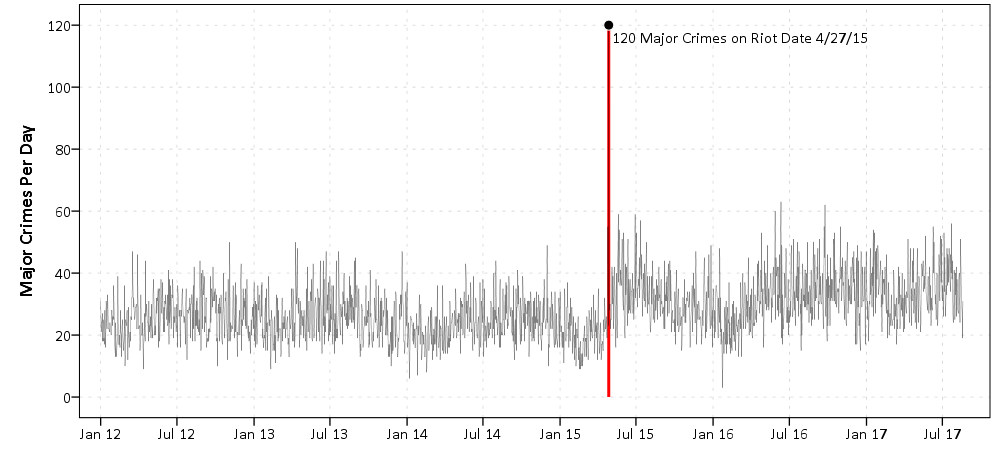The bars in Pete’s graph are not the easiest way to visualize the trend. Here making the line thin and lighter grey also helps.

The way to analyze this data is using an interrupted time series analysis. I am not going to go through all of those details, but for those interested I would suggest picking up David McDowell’s little green book, Interrupted Time Series Analysis, for a walkthrough. One of the first steps though is to figure out the ARIMA structure, which you do by examining the auto-correlation function. Here is that ACF for this crime data.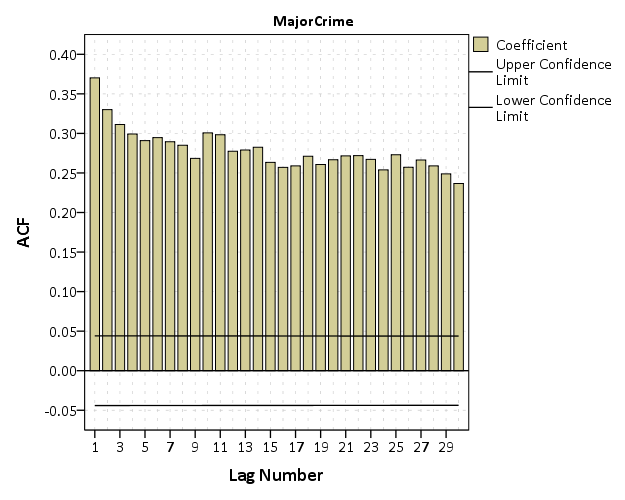You can see that it is positive and stays quite consistent. This is indicative of a moving average model. It does not show the geometric decay of an auto-regressive process, nor is the autocorrelation anywhere near 1, which you would expect for an integrated process. Also the partial autocorrelation plot shows the geometric decay, which is again consistent with a moving average model. See my note at the bottom, how this interpretation was wrong! (Via David Greenberg sent me a note.)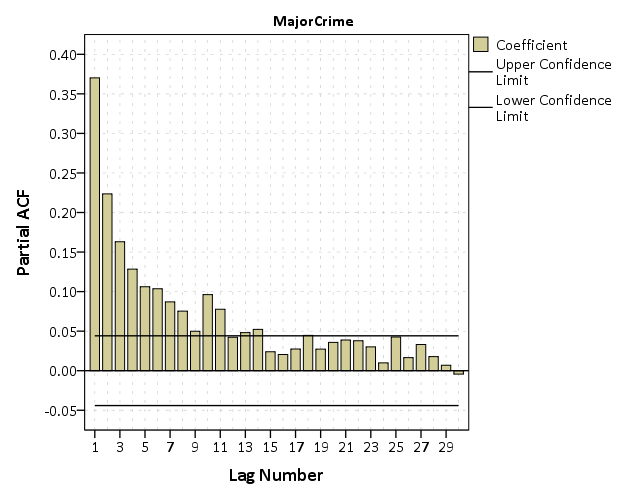Although it is typical to analyze crime counts as a Poisson model, I often like to use linear models. Coefficients are much easier to interpret. Here the distribution of the counts is high enough I am ok using a linear interrupted ARIMA model.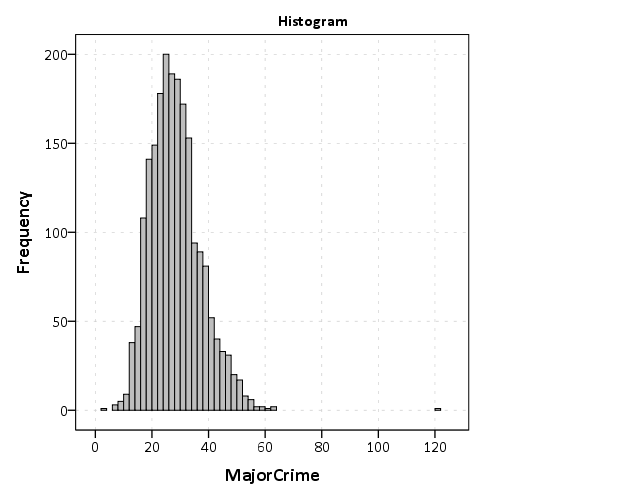So I estimated an interrupted time series model. I include a dummy variable term that equals 1 as of 4/27/15 and after, and equals 0 before. That variable is labeled `PostRiot`. I then have dummy variables for each month of the year (M1, M2, …., M11) and days of the week (D1,D2,….D6). The ARIMA model I estimate then is (0,0,7), with a constant. Here is that estimate.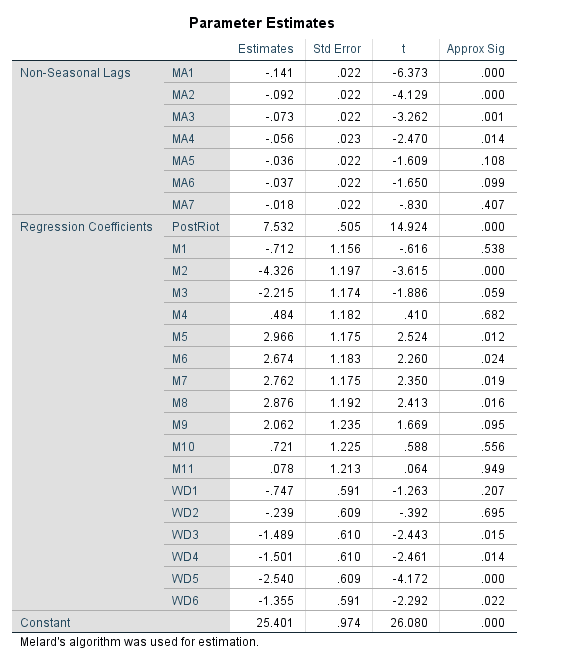So we get an estimate that post riot, major crimes have increased by around 7.5 per day. This is pretty similar to what you get when you just look at the daily mean pre-post riot, so it isn’t really any weird artifact of my modeling strategy. Pre-riot it is under 25 per day, and post it is over 32 per day.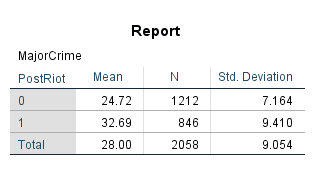This result is pretty robust across different model specifications. Dropping the constant term results in a larger post riot estimate (over 10). Inclusion of fewer or more MA terms (as well as seasonal MA terms for 7 days) does not change the estimate. Inclusion of the monthly or day of week dummy variables does not make a difference in the estimate. Changing the outlier value on 4/27/15 to a lower value (here I used the pre-mean, 24) does reduce the estimate slightly, but only to 7.2.

There is a bit of residual autocorrelation I was never able to get rid of, but it is fairly small, with the highest autocorrelation of only about 0.06.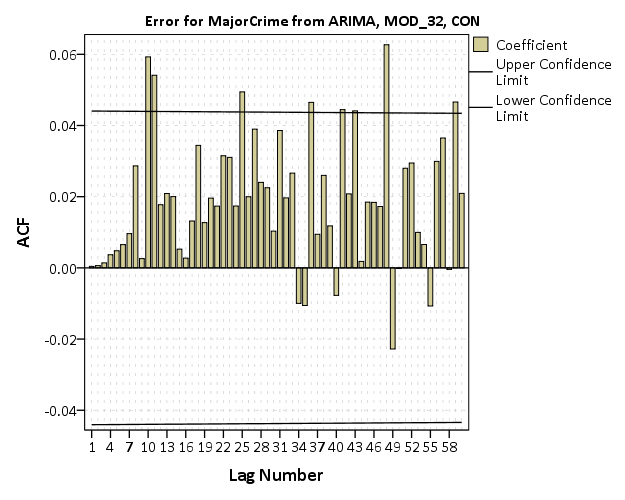Here is the SPSS code to reproduce the Baltimore graphs and ARIMA analysis.

As a note, while Pete believes this is a result of depolicing (i.e. Baltimore officers being less proactive) the evidence for that hypothesis is not necessarily confirmed by this analysis. See Stephen Morgan’s analysis on crime and arrests, although I think proactive street stops should likely also be included in such an analysis.

This Baltimore data just shows a bump up in the series, but investigating homicides in Chicago (here at the monthly level) it looks to me like an upward trend post the McDonald shooting. This graph is at the monthly level.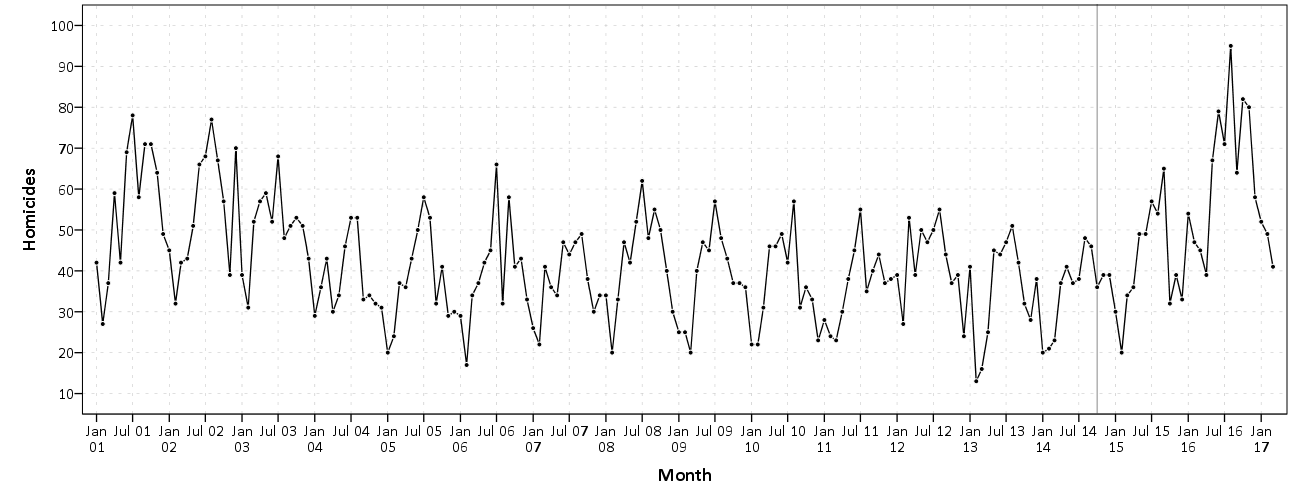I have some other work on Chicago homicide geographic patterns going back quite a long time I can hopefully share soon!

I will need to update the Baltimore analysis to look at just homicides as well. Pete shows a similar bump in his charts when just examining homicides.

For additional resources for folks interested in examining crime over time, I would suggest checking out my article, Monitoring volatile homicide trends across U.S. cities, as well as Tables and Graphs for Monitoring Crime Patterns. I’m doing a workshop at the upcoming International Association for Crime Analysts conference on how to recreate such graphs in Excel.

David Greenberg sent me an email  to note my interpretation of the ACF plots was wrong – and that a moving average process should only have a spike, and not show the slow decay. He is right, and so I updated the interrupted ARIMA models to include higher order AR terms instead of MA terms. The final model I settled on was (5,0,0) — I kept adding higher order AR terms until the AR coefficients were not statistically significant. For these models I still included a constant.

For the model that includes the outlier riot count, it results in an estimate that the riot increased these crimes by 7.5 per day, with a standard error of 0.5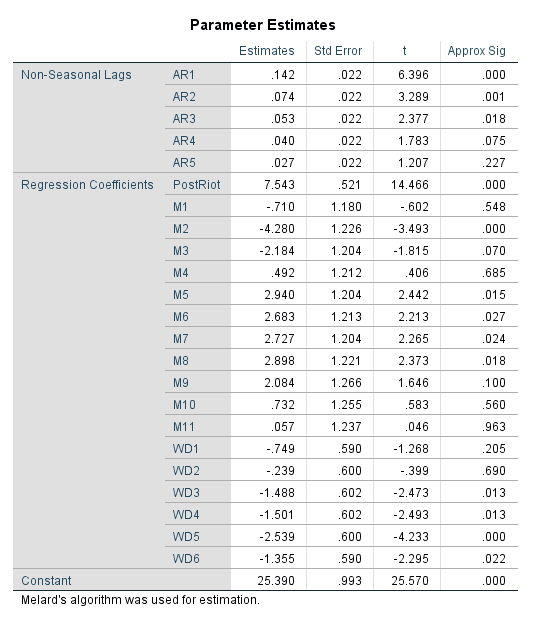This model has no residual auto-correlation until you get up to very high lags. Here is a table of the Box-Ljung stats for up to 60 lags.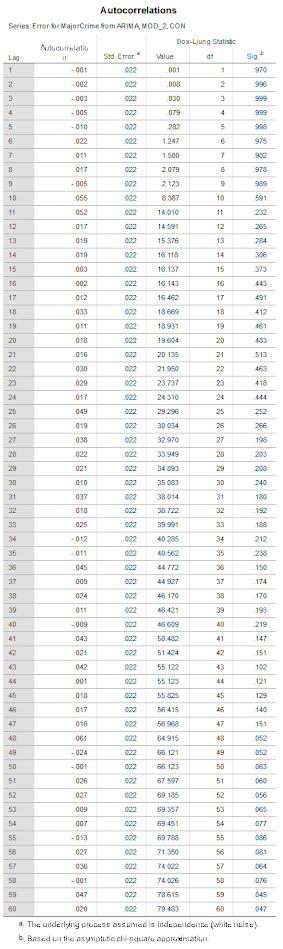Estimating the same ARIMA model with the outlier value changed to 24, the post riot estimate is still over 7.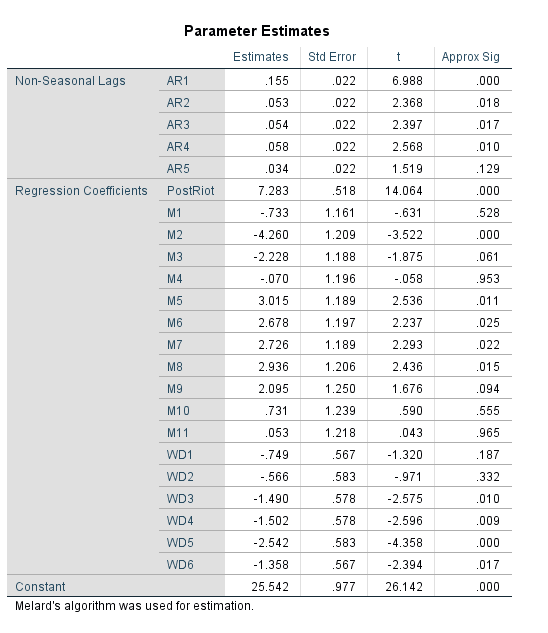Subsequently the post-riot increase estimate is pretty robust across these different ARIMA model settings. The lowest estimate I was able to get was a post mean increase of 5 when not including an intercept and not including the outlier crime counts on the riot date. So I think this result holds up pretty well to a bit of scrutiny.

# IACA Conference 2017 workshop: Monitoring temporal crime trends for outliers (Excel)

This fall at the International Association of Crime Analysts conference I am doing a workshop, Monitoring temporal crime trends for outliers: A workshop using Excel. If you can’t wait (or are not going) I have all my materials already prepared, which you can download here. That includes a walkthrough of my talk/tutorial, as well as a finished Excel workbook. It is basically a workshop to go with my paper, Tables and graphs for monitoring temporal crime trends: Translating theory into practical crime analysis advice.

For some preview, I will show how to make a weekly smoothed chart with error bands: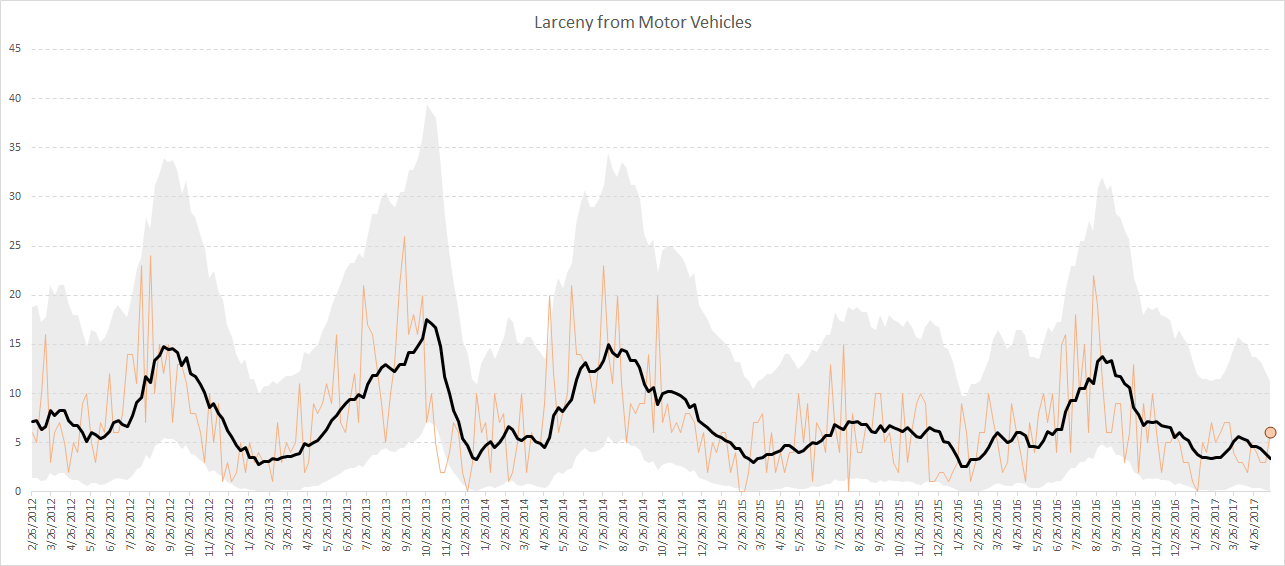As well as a monthly seasonal chart: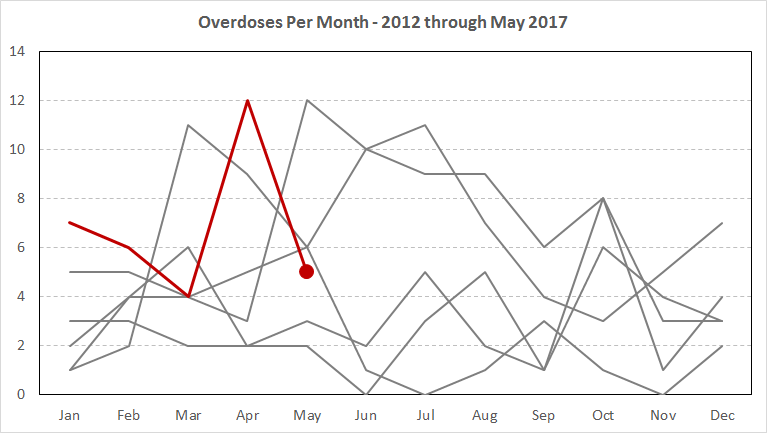I use Excel not because I think it is the best tool, but mainly because I think it is the most popular among crime analysts. In the end I just care about getting the job done! (Although I’ve given reasons why I think Excel is more painful than any statistical program.) Even though it is harder to make small multiple charts in Excel, I show how to make these charts using pivot tables and filters, so watching them auto-update when you update the filter is pretty cool.

For those with SPSS I have already illustrated how to make similar charts in SPSS here. You could of course replicate that in R or Stata or whatever if you wanted.

I am on the preliminary schedule currently for Tuesday, September 12th at 13:30 to 14:45. I will be in New Orleans on the 11th, 12th and 13th, so if you want to meet always feel free to send an email to set up a time.

# Weekly and monthly graphs for monitoring crime patterns (SPSS)

I was recently asked for some code to show how I created the charts in my paper, Tables and Graphs for Monitoring Crime Patterns (Pre-print can be seen here).

I cannot share the data used in the paper, but I can replicate them with some other public data. I will use calls for service publicly available from Burlington, VT to illustrate them.

The idea behind these time-series charts are not for forecasting, but to identify anomalous patterns – such as recent spikes in the data. (So they are more in line with the ideas behind control charts.) Often even in big jurisdictions, one prolific offender can cause a spike in crimes over a week or a month. They are also good to check more general trends as well, to see if crimes have had more slight, but longer term trends up or down.

For a preview, we will be making a weekly time series chart: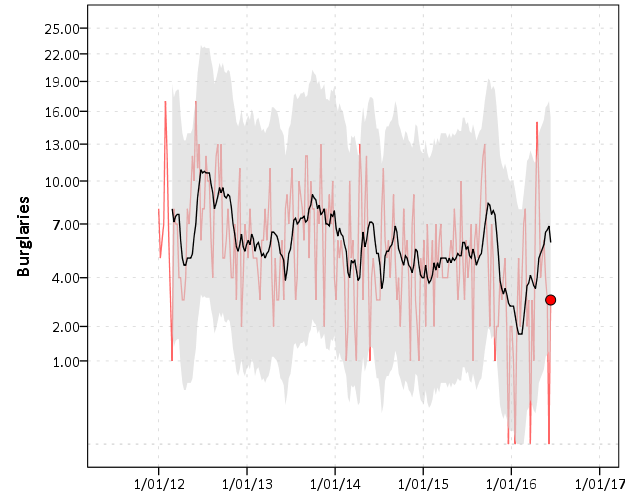In the weekly chart the red line is the actual data, the black line is the average of the prior 8 weeks, and the grey band is a Poisson confidence interval around that prior moving average. The red dot is the most recent week.

And we will also be making a monthly seasonal chart: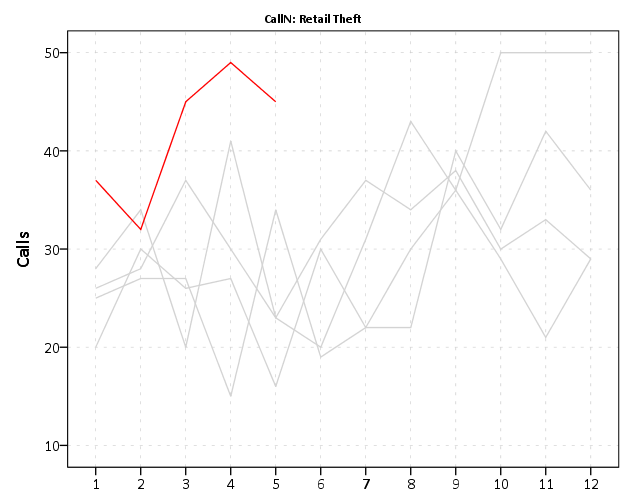The red line is the counts of calls per month in the current year, and the lighter grey lines are prior years (here going back to 2012).

So to start, I saved the 2012 through currently 6/20/2016 calls for service data as a csv file. And here is the code to read in that incident level data.

``````*Change this to where the csv file is located on your machine.
FILE HANDLE data /NAME = "C:\Users\andrew.wheeler\Dropbox\Documents\BLOG\Tables_Graphs".
GET DATA  /TYPE=TXT
/FILE="data\Calls_for_Service_Dashboard_data.csv"
/ENCODING='UTF8'
/DELCASE=LINE
/DELIMITERS=","
/QUALIFIER='"'
/ARRANGEMENT=DELIMITED
/FIRSTCASE=2
/DATATYPEMIN PERCENTAGE=95.0
/VARIABLES=
AlcoholRelated AUTO
Area AUTO
CallDateTime AUTO
CallType AUTO
Domv AUTO
DayofWeek AUTO
DrugRelated AUTO
EndDateTime AUTO
GeneralTimeofDay AUTO
IncidentNumber AUTO
LocationType AUTO
MentalHealthRelated AUTO
MethodofEntry AUTO
Month AUTO
PointofEntry AUTO
StartDateTime AUTO
Street AUTO
Team AUTO
Year AUTO
/MAP.
CACHE.
EXECUTE.
DATASET NAME CFS.``````

First I will be making the weekly chart. What I did when I was working as an analyst was make a chart that showed the recent weekly trends and to identify if the prior week was higher than you might expect it to be. The weekly patterns can be quite volatile though, so I smoothed the data based on the average of the prior eight weeks, and calculated a confidence interval around that average count (based on the Poisson distribution).

As a start, we are going to turn our date variable, `CallDateTime`, into an SPSS date variable (it gets read in as a string, AM/PM in date-times are so annoying!). Then we are going to calculate the number of days since some baseline – here it is 1/1/2012, which is Sunday. Then we are going to calculate the weeks since that Sunday. Lastly we select out the most recent week, as it is not a full week.

``````*Days since 1/1/2012.
COMPUTE #Sp = CHAR.INDEX(CallDateTime," ").
COMPUTE CallDate = NUMBER(CHAR.SUBSTR(CallDateTime,1,#Sp),ADATE10).
COMPUTE Days = DATEDIFF(CallDate,DATE.MDY(1,1,2012),"DAYS").
COMPUTE Weeks = TRUNC( (Days-1)/7 ).
FREQ Weeks /FORMAT = NOTABLE /STATISTICS = MIN MAX.
SELECT IF Weeks < 233.``````

Here I do weeks since a particular date, since if you do `XDATE.WEEK` you can have not full weeks. The magic number 233 can be replaced by sometime like `SELECT IF Weeks < (\$TIME - 3*24*60*60).` if you know you will be running the syntax on a set date, such as when you do a production job. (Another way is to use `AGGREGATE` to figure out the latest date in the dataset.)

Next what I do is that when you use `AGGREGATE` in SPSS, there can be missing weeks with zeroes, which will mess up our charts. There end up being 22 different call-types in the Burlington data, so I make a base dataset (named `WeekFull`) that has all call types for each week. Then I aggregate the original calls for service dataset to CallType and Week, and then I merge the later into the former. Finally I then recode the missings intos zeroes.

``````*Make sure I have a full set in the aggregate.
FREQ CallType.
AUTORECODE CallType /INTO CallN.
*22 categories, may want to collapse a few together.
INPUT PROGRAM.
LOOP #Weeks = 0 TO 232.
LOOP #Calls = 1 TO 22.
COMPUTE CallN = #Calls.
COMPUTE Weeks = #Weeks.
END CASE.
END LOOP.
END LOOP.
END FILE.
END INPUT PROGRAM.
DATASET NAME WeekFull.

*Aggregate number of tickets to weeks.
DATASET ACTIVATE CFS.
DATASET DECLARE WeekCalls.
AGGREGATE OUTFILE='WeekCalls'
/BREAK Weeks CallN
/CallType = FIRST(CallType)
/TotCalls = N.

*Merge Into WeekFull.
DATASET ACTIVATE WeekFull.
MATCH FILES FILE = *
/FILE = 'WeekCalls'
/BY Weeks CallN.
DATASET CLOSE WeekCalls.
*Missing are zero cases.
RECODE TotCalls (SYSMIS = 0)(ELSE = COPY).``````

Now we are ready to calculate our statistics and make our charts. First we create a date variable that represents the beginning of the week (for our charts later on.) Then I use `SPLIT FILE` and `CREATE` to calculate the prior moving average only within individiual call types. The last part of the code calculates a confidence interval around prior moving average, and assumes the data is Poisson distributed. (More discussion of this is in my academic paper.)

``````DATASET ACTIVATE WeekFull.
COMPUTE WeekBeg = DATESUM(DATE.MDY(1,1,2012),(Weeks*7),"DAYS").

*Moving average of prior 8 weeks.
SORT CASES BY CallN Weeks.
SPLIT FILE BY CallN.
CREATE MovAv = PMA(TotCalls,8).
*Calculating the plus minus 3 Poisson intervals.
COMPUTE #In = (-3/2 + SQRT(MovAv)).
DO IF #In >= 0.
COMPUTE LowInt = #In**2.
ELSE.
COMPUTE LowInt = 0.
END IF.
COMPUTE HighInt = (3/2 + SQRT(MovAv))**2.
EXECUTE.``````

If you rather use the inverse of the Poisson distribution I have notes in the code at the end to do that, but they are pretty similar in my experience. You also might consider (as I mention in the paper), rounding fractional values for the `LowInt` down to zero as well.

Now we are ready to make our charts. The last data manipulation is to just put a flag in the file for the very last week (which will be marked with a large red circle). I use `EXECUTE` before the chart just to make sure the variable is available. Finally I keep the `SPLIT FILE` on, which produces 22 charts, one for each call type.

``````IF Weeks = 232 FinCount = TotCalls.
EXECUTE.

*Do a quick look over all of them.
GGRAPH
/GRAPHDATASET NAME="graphdataset" VARIABLES=WeekBeg TotCalls MovAv LowInt HighInt FinCount MISSING=VARIABLEWISE
/GRAPHSPEC SOURCE=INLINE.
BEGIN GPL
SOURCE: s=userSource(id("graphdataset"))
DATA: WeekBeg=col(source(s), name("WeekBeg"))
DATA: TotCalls=col(source(s), name("TotCalls"))
DATA: MovAv=col(source(s), name("MovAv"))
DATA: LowInt=col(source(s), name("LowInt"))
DATA: HighInt=col(source(s), name("HighInt"))
DATA: FinCount=col(source(s), name("FinCount"))
SCALE: pow(dim(2), exponent(0.5))
GUIDE: axis(dim(1))
GUIDE: axis(dim(2), label("Crime Count"))
ELEMENT: line(position(WeekBeg*TotCalls), color(color.red), transparency(transparency."0.4"))
transparency.interior(transparency."0.4"), transparency.exterior(transparency."1"))
ELEMENT: line(position(WeekBeg*MovAv))
ELEMENT: point(position(WeekBeg*FinCount), color.interior(color.red), size(size."10"))
END GPL.
SPLIT FILE OFF.``````

This is good for the analyst, I can monitor many series. Here is an example the procedure produces for mental health calls:The current value is within the confidence band, so it is not alarmingly high. But we can see that they have been trending up over the past few years. Plotting on the square root scale makes the Poisson count data have the same variance, but a nice thing about the SPLIT FILE approach is that SPSS does smart Y axis ranges for each individual call type.

You can update this to make plots for individual crimes, and here I stuff four different crime types into a small multiple plot. I use a `TEMPORARY` and `SELECT IF` statement before the `GGRAPH` code to select out the crime types I am interested in.

``````FORMATS TotCalls MovAv LowInt HighInt FinCount (F3.0).
TEMPORARY.
SELECT IF ANY(CallN,3,10,13,17).
GGRAPH
/GRAPHDATASET NAME="graphdataset" VARIABLES=WeekBeg TotCalls MovAv LowInt HighInt FinCount CallN MISSING=VARIABLEWISE
/GRAPHSPEC SOURCE=INLINE.
BEGIN GPL
PAGE: begin(scale(900px,600px))
SOURCE: s=userSource(id("graphdataset"))
DATA: WeekBeg=col(source(s), name("WeekBeg"))
DATA: TotCalls=col(source(s), name("TotCalls"))
DATA: MovAv=col(source(s), name("MovAv"))
DATA: LowInt=col(source(s), name("LowInt"))
DATA: HighInt=col(source(s), name("HighInt"))
DATA: FinCount=col(source(s), name("FinCount"))
DATA: CallN=col(source(s), name("CallN"), unit.category())
COORD: rect(dim(1,2), wrap())
SCALE: pow(dim(2), exponent(0.5))
GUIDE: axis(dim(1))
GUIDE: axis(dim(2), start(1), delta(3))
GUIDE: axis(dim(3), opposite())
GUIDE: form.line(position(*,0),color(color.lightgrey),shape(shape.half_dash))
ELEMENT: line(position(WeekBeg*TotCalls*CallN), color(color.red), transparency(transparency."0.4"))
transparency.interior(transparency."0.4"), transparency.exterior(transparency."1"))
ELEMENT: line(position(WeekBeg*MovAv*CallN))
ELEMENT: point(position(WeekBeg*FinCount*CallN), color.interior(color.red), size(size."10"))
PAGE: end()
END GPL.
EXECUTE.``````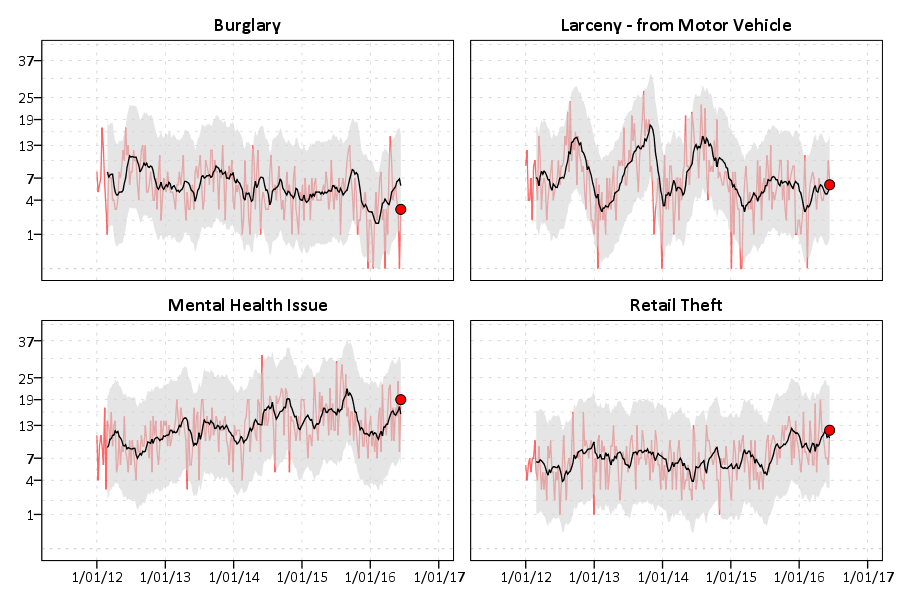You could do more fancy time-series models to create the confidence bands or identify the outliers, (exponential smoothing would be similar to just the prior moving average I show) but this ad-hoc approach worked well in my case. (I wanted to make more fancy models, but I did not let the perfect be the enemy of the good to get at least this done when I was employed as a crime analyst.)

Now we can move onto making our monthly chart. These weekly charts are sometimes hard to visualize with highly seasonal data. So what this chart does is gives each year a new line. Instead of drawing error bars, the past years data show the typical variation. It is then easy to see seasonal ups-and-downs, as well as if the latest month is an outlier.

Getting back to the code — I activate the original calls for service database and then close the Weekly database. Then it is much the same as for weeks, but here I just use calendar months and match to a full expanded set of calls types and months over the period. (I do not care about normalizing months, it is ok that February is only 28 days).

``````DATASET ACTIVATE CFS.
DATASET CLOSE WeekFull.

COMPUTE Month = XDATE.MONTH(CallDate).
COMPUTE Year = XDATE.YEAR(CallDate).

DATASET DECLARE AggMonth.
AGGREGATE OUTFILE = 'AggMonth'
/BREAK Year Month CallN
/MonthCalls = N.

INPUT PROGRAM.
LOOP #y = 2012 TO 2016.
LOOP #m = 1 TO 12.
LOOP #call = 1 TO 22.
COMPUTE CallN = #call.
COMPUTE Year = #y.
COMPUTE Month = #m.
END CASE.
END LOOP.
END LOOP.
END LOOP.
END FILE.
END INPUT PROGRAM.
DATASET NAME MonthAll.

MATCH FILES FILE = *
/FILE = 'AggMonth'
/BY Year Month CallN.
DATASET CLOSE AggMonth.``````

Next I select out the most recent month of the date (June 2016) since it is not a full month. (When I originally made these charts I would normalize to days and extrapolate out for my monthly meeting. These forecasts were terrible though, even only extrapolating two weeks, so I stopped doing them.) Then I calculate a variable called `Current` – this will flag the most recent year to be red in the chart.

``````COMPUTE MoYr = DATE.MDY(Month,1,Year).
FORMATS MoYr (MOYR6) Year (F4.0) Month (F2.0).
SELECT IF MoYr < DATE.MDY(6,1,2016).
RECODE MonthCalls (SYSMIS = 0)(ELSE = COPY).

*Making current year red.
COMPUTE Current = (Year = 2016).
FORMATS Current (F1.0).

SORT CASES BY CallN MoYr.
SPLIT FILE BY CallN.

*Same thing with the split file.
GGRAPH
/GRAPHDATASET NAME="graphdataset" VARIABLES=Month MonthCalls Current Year
/GRAPHSPEC SOURCE=INLINE.
BEGIN GPL
SOURCE: s=userSource(id("graphdataset"))
DATA: Month=col(source(s), name("Month"), unit.category())
DATA: MonthCalls=col(source(s), name("MonthCalls"))
DATA: Current=col(source(s), name("Current"), unit.category())
DATA: Year=col(source(s), name("Year"), unit.category())
GUIDE: axis(dim(1))
GUIDE: axis(dim(2), label("Calls"), start(0))
GUIDE: legend(aesthetic(aesthetic.color.interior), null())
SCALE: cat(aesthetic(aesthetic.color.interior), map(("0",color.lightgrey),("1",color.red)))
ELEMENT: line(position(Month*MonthCalls), color.interior(Current), split(Year))
END GPL.``````

You can again customize this to be individual charts for particular crimes or small multiples. You can see in the example at the beginning of the post Retail thefts are high for March, April and May. I was interested to examine overdoses, as the northeast (and many parts of the US) are having a problem with heroin at the moment. In the weekly charts they are so low of counts it is hard to see any trends though.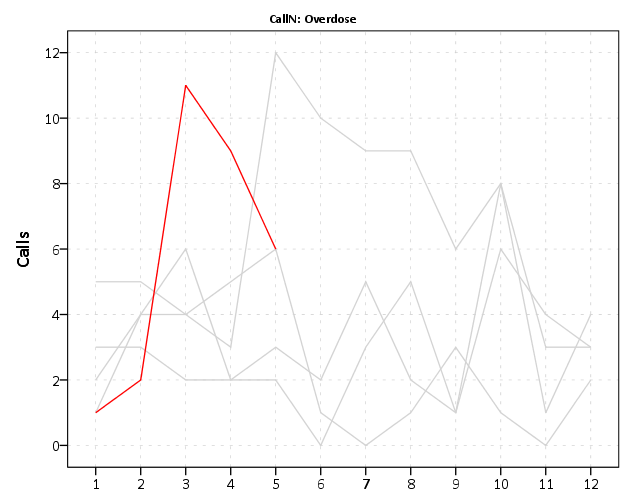We can see that overdoses were high in March. The other highest line are months in 2015, so it looks like a problem here in Burlington, but it started around a year ago.

For low counts of crime (say under 20 per month) seasonality tends to be hard to spot. For crimes more frequent though you can often see pits and peaks in summer and winter. It is not universal that crimes increase in the summer though. For ordinance violations (and ditto for Noise complaints) we can see a pretty clear peak in September. (I don’t know why that is, there is likely some logical explanation for it though.)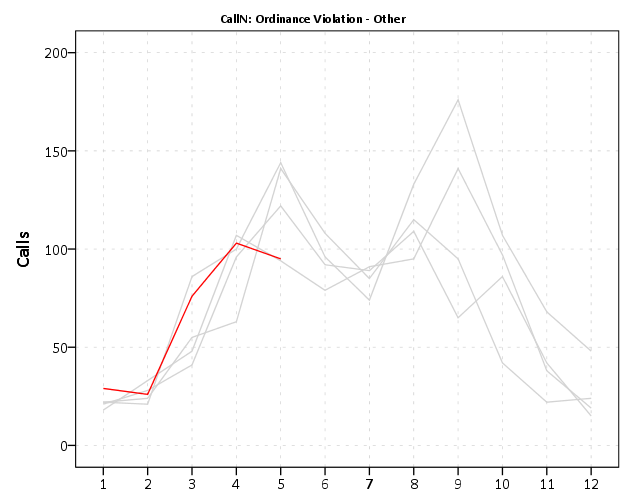My main motivation to promote these is to replace terrible CompStat tables of year-over-year percent changes. All of these patterns I’ve shown are near impossible to tell from tables of counts per month.

Finally if you want to export your images to place into another report, you can use:

``OUTPUT EXPORT /PNG IMAGEROOT = "data\TimeGraphs.png".``

PNG please – simple vector graphics like these should definately not be exported as jpegs.

Here is a link to the full set of syntax and the csv data to follow along. I submitted to doing an hour long training session at the upcoming IACA conference on this, so hopefully that gets funded and I can go into this some more.

# Estimating group based trajectory models using SPSS and R

For a project I have been estimating group based trajectory models for counts of crime at micro places. Synonymous with the trajectory models David Weisburd and colleagues estimated for street segments in Seattle. Here I will show how using SPSS and the R package `crimCV` one can estimate similar group based trajectory models. Here is the `crimCV` package help and here is a working paper by the authors on the methodology. The package specifically estimates a zero inflated poisson model with the options to make the 0-1 part and/or the count part have quadratic or cubic terms – and of course allows you specify the number of mixture groups to search for.

So first lets make a small set of fake data to work with. I will make 100 observations with 5 time points. The trajectories are three separate groups (with no zero inflation).

``````*Make Fake data.
SET SEED 10.
INPUT PROGRAM.
LOOP Id = 1 TO 100.
END CASE.
END LOOP.
END FILE.
END INPUT PROGRAM.
DATASET NAME OrigData.
*Make 3 fake trajectory profiles.
VECTOR Count(5,F3.0).
DO REPEAT Count = Count1 TO Count5 /#iter = 1 TO 5.
COMPUTE #i = #iter - 3.
COMPUTE #ii  = #i**2.
COMPUTE #iii = #i**3.
DO IF Id <= 30.
COMPUTE #P    = 10 + #i*0.3 + #ii*-0.1 + #iii*0.05.
COMPUTE Count = RV.POISSON(#P).
ELSE IF Id <=60.
COMPUTE #P    =  5 + #i*-0.8 + #ii*0.3 + #iii*0.05.
COMPUTE Count = RV.POISSON(#P).
ELSE.
COMPUTE #P    =  4 + #i*0.8 + #ii*-0.5 + #iii*0.
COMPUTE Count = RV.POISSON(#P).
END IF.
END REPEAT.
FORMATS Id Count1 TO Count5 (F3.0).
EXECUTE.``````

Note The `crimCV` package wants the data to be wide format for the models, that is each separate time point in its own column. Now we can call R code using `BEGIN PROGRAM R` to recreate the wide SPSS dataset in an R data frame.

``````*Recreate SPSS data in R data frame.
BEGIN PROGRAM R.
casedata <- spssdata.GetDataFromSPSS(variables=c("Id","Count1","Count2",
"Count3","Count4","Count5")) #grab data from SPSS
casedataMat <- as.matrix(casedata[,2:6]) #turn into matrix
#summary(casedata) #check contents
#casedataMat[1:10,1:5]
END PROGRAM.``````

Now to fit one model with 3 groups (without calculating the cross validation statistics) the code would be as simple as:

``````*Example estimating model with 3 groups and no CV.
BEGIN PROGRAM R.
library(crimCV)
crimCV(casedataMat,3,rcv=FALSE,dpolyp=3,dpolyl=3)
END PROGRAM.``````

But when we are estimating these group based trajectory models we don’t know the number of groups in advance. So typically one progressively fits more groups and then uses model selection criteria to pick the mixture solution that best fits the data. Here is a loop I created to successively estimate models with more groups and stuffs the models results in a list. It also makes a separate data frame that saves the model fit statistics, so you can see which solution fits the best (at least based on these statistics). Here I loop through estimates of 1 through 4 groups (this takes about 2 minutes in this example). Be warned – here are some bad programming practices in R (the for loops are defensible, but growing the lists within the loop is not – they are small though in my application and I am lazy).

``````*looping through models 1 through 4.
BEGIN PROGRAM R.
results <- c()  #initializing a set of empty lists to store the seperate models
measures <- data.frame(cbind(groups=c(),llike=c(),AIC=c(),BIC=c(),CVE=c())) #nicer dataframe to check out model
#model selection diagnostics
max <- 4 #this is the number of grouping solutions to check

#looping through models
for (i in 1:max){
model <- crimCV(casedataMat,i,rcv=TRUE,dpolyp=3,dpolyl=3)
results <- c(results, list(model))
measures <- rbind(measures,data.frame(cbind(groups=i,llike=model\$llike,
AIC=model\$AIC,BIC=model\$BIC,CVE=model\$cv)))
#save(measures,results,file=paste0("Traj",as.character(i),".RData")) #save result
}
#table of the model results
measures
END PROGRAM.``````

In my actual application the groups take a long time to estimate, so I have the commented line saving the resulting list in a file. Also if the model does not converge it breaks the loop. So here we see that the mixture with 3 groups is the best fit according to the CVE error, but the 2 group solution would be chosen by AIC or BIC criteria. Just for this tutorial I will stick with the 3 group solution. We can plot the predicted trajectories right within R by selecting the nested list.

``````*plot best fitting model.
BEGIN PROGRAM R.
plot(results[])
#getAnywhere(plot.dmZIPt) #this is the underlying code
END PROGRAM.``````

Now the particular object that stores the probabilities is within the `gwt` attribute, so we can transform this to a data frame, merge in the unique identifier, and then use the `STATS GET R` command to grab the resulting R data frame back into an SPSS dataset.

``````*Grab probabiltiies back SPSS dataset.
BEGIN PROGRAM R.
myModel <- results[] #grab model
myDF <- data.frame(myModel\$gwt) #probabilites into dataframe
myDF\$Id <- casedata\$Id #add in Id
END PROGRAM.
*back into SPSS dataset.
STATS GET R FILE=* /GET DATAFRAME=myDF DATASET=TrajProb.``````

Then we can merge this R data frame into SPSS. After that, we can classify the observations into groups based on the maximum posterior probability of belonging to a particular group.

``````*Merge into original dataset, and the assign groups.
DATASET ACTIVATE OrigData.
MATCH FILES FILE = *
/FILE = 'TrajProb'
/BY ID
/DROP row.names.
DATASET CLOSE TrajProb.
*Assign group based on highest prob.
*If tied last group wins.
VECTOR X = X1 TO X3.
COMPUTE #MaxProb = MAX(X1 TO X3).
LOOP #i = 1 TO 3.
IF X(#i) = #MaxProb Group = #i.
END LOOP.
FORMATS Group (F1.0).``````

Part of the motivation for doing this is not only to replicate some of the work of Weisburd and company, but that it has utility for identifying long term hot spots. Part of what Weisburd (and similarly I am) finding is that crime at small places is pretty stable over long periods of time. So we don’t need to make up to date hotspots to allocate police resources, but are probably better off looking at crime over much longer periods to identify places for targeted strategies. Trajectory models are a great tool to help identify those long term high crime places, same as geographic clustering is a great tool to help identify crime hot spots.